# Math Olympiad Test: Quadratic Equations- 2

Test Description

## 15 Questions MCQ Test International Mathematics Olympiad (IMO) for Class 10 | Math Olympiad Test: Quadratic Equations- 2

Math Olympiad Test: Quadratic Equations- 2 for Class 10 2022 is part of International Mathematics Olympiad (IMO) for Class 10 preparation. The Math Olympiad Test: Quadratic Equations- 2 questions and answers have been prepared according to the Class 10 exam syllabus.The Math Olympiad Test: Quadratic Equations- 2 MCQs are made for Class 10 2022 Exam. Find important definitions, questions, notes, meanings, examples, exercises, MCQs and online tests for Math Olympiad Test: Quadratic Equations- 2 below.
Solutions of Math Olympiad Test: Quadratic Equations- 2 questions in English are available as part of our International Mathematics Olympiad (IMO) for Class 10 for Class 10 & Math Olympiad Test: Quadratic Equations- 2 solutions in Hindi for International Mathematics Olympiad (IMO) for Class 10 course. Download more important topics, notes, lectures and mock test series for Class 10 Exam by signing up for free. Attempt Math Olympiad Test: Quadratic Equations- 2 | 15 questions in 15 minutes | Mock test for Class 10 preparation | Free important questions MCQ to study International Mathematics Olympiad (IMO) for Class 10 for Class 10 Exam | Download free PDF with solutions
 1 Crore+ students have signed up on EduRev. Have you?
Math Olympiad Test: Quadratic Equations- 2 - Question 1

### Find the value of x. (Be sure to give all solutions) x2 - 6 = 138

Detailed Solution for Math Olympiad Test: Quadratic Equations- 2 - Question 1

Simplifying x2 + -6 = 138
Reorder the terms: -6 + x2 = 138
Solving -6 + x2 = 138
Solving for variable 'x'
Move all terms containing x to the left, all other terms to the right
Add '6' to each side of the equation
-6 + 6 + x2 = 138 + 6
Combine like terms: -6 + 6 = 0
0 + x2 = 138 + 6
x2 = 138 + 6
Combine like terms: 138 + 6 = 144
x2 = 144
Simplifying x2 = 144
Take the square root of each side:
x = {-12, 12}

Math Olympiad Test: Quadratic Equations- 2 - Question 2

### Find the values for x for the following equation. x2 + 4x - 32 = 0

Detailed Solution for Math Olympiad Test: Quadratic Equations- 2 - Question 2

This equation is written in standard form and it is set equal to 0. Therefore, I know that the 2 methods for solving are factoring and using the quadratic equation.
As I browse the answers, it looks like the values for x are integers, so I must be able to factor. Therefore, this will be the easiest method.
I need to find two integers whose product is -32 and whose sum is 4. (8 & -4).
Don’t make the mistake of assuming these are your answers!!!
(x + 8) (x - 4) = 0 Rewrite as factors
x + 8 = 0 x - 4 = 0
Set each factor equal to 0
x + 8 - 8 = 0 - 8
x - 4 + 4 = 0 + 4
x = - 8,  x = 4

Math Olympiad Test: Quadratic Equations- 2 - Question 3

### Find the values of y for the following equation: 2y2 - 5y + 2 = 5

Detailed Solution for Math Olympiad Test: Quadratic Equations- 2 - Question 3

In order to find the values for y, we must set the equation equal to 0 by subtracting 5 from both sides.
2y2 - 5y + 2 - 5 = 5 - 5
Subtract 5 from both sides
2y2 - 5y - 3 = 0
Now the equation is set equal to 0.
As I take a look at the answers, it looks like this equation can be factored. Let’s try!
(2y + 1) (y - 3) = 0 Factor the equation
2y + 1 = 0   y - 3 = 0
Set each factor equal to 0
2y + 1 - 1 = 0 - 1     y - 3 + 3 = 0 + 3
2y = -1   y = 3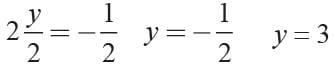Math Olympiad Test: Quadratic Equations- 2 - Question 4

A ball is shot from a cannon into the air with an upward velocity of 36 ft/sec. The equation that gives the height (h) of the ball at any time (t) is: h(t) = -16t2 + 36t + 1.5. Find the maximum height attained by the ball.

Detailed Solution for Math Olympiad Test: Quadratic Equations- 2 - Question 4

In order to find the maximum height of the ball, I would need to find the y-coordinate of the vertex.
Vertex formula: x = - b/2a
where: a = -16 b = 36 c = 1.5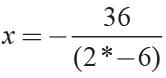x = 1.125
Now substitute 1.125 for t into the equation and solve for h(t). H(t) = -16 (1.125)2 + 36 (1.125) + 1.5
H(t) = 21.75

Math Olympiad Test: Quadratic Equations- 2 - Question 5

If a < 0, then function f(x) = ax2 + bx + c has a maximum value at

Detailed Solution for Math Olympiad Test: Quadratic Equations- 2 - Question 5

The graph of a quadratic equation is parabola.
If a < 0, then the parabola opens downward. If the parabola opens downward, then the vertex is the point whose y-value is the maximum value of f.

Math Olympiad Test: Quadratic Equations- 2 - Question 6

Quadratic function is defined as

Detailed Solution for Math Olympiad Test: Quadratic Equations- 2 - Question 6

Quadratic function is a function that can be described by an equation of the form fxx = ax2 + bx + c, where a ≠ 0.
In a quadratic function, the greatest power of the variable is 2. The graph of a quadratic function is a parabola.

Math Olympiad Test: Quadratic Equations- 2 - Question 7

If α  and β are the roots of 3x2 + 8x +2 = 0 then what is the value α2 + β2?

Detailed Solution for Math Olympiad Test: Quadratic Equations- 2 - Question 7

Given equation is
3x2 + 8x + 2 = 0
and α, β are its roots then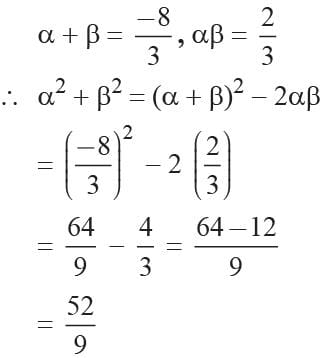Math Olympiad Test: Quadratic Equations- 2 - Question 8

If the sum of roots of the equation Kx2 + 2x + 3K = 0 is equal to their product, then the value of K is

Detailed Solution for Math Olympiad Test: Quadratic Equations- 2 - Question 8

Here Kx2 + 2x + 3K = 0
then given Sum of roots = Product of roots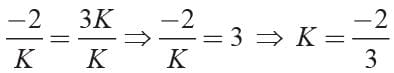Math Olympiad Test: Quadratic Equations- 2 - Question 9

The roots of a quadratic equation are 7 and -3, then what is the equation?

Detailed Solution for Math Olympiad Test: Quadratic Equations- 2 - Question 9

The required equation is
x2 - (Sum of roots)x + Product of roots = 0
⇒ x2 - [7 + (- 3)]x + (7) (- 3) = 0
⇒ x2 - 4x - 21 = 0

Math Olympiad Test: Quadratic Equations- 2 - Question 10

If one root of the equation x2 + px + 12 = 0 is 4, while the equation x2 + px + q = 0 has equal roots then the value of q is

Detailed Solution for Math Olympiad Test: Quadratic Equations- 2 - Question 10

Here x2 + px + q = 0 has one root a = 4
∴ 42 + b4 + 12 = 0
⇒ b = -7
Now the equation x2 - 7x + q = 0 has equal roots
(-7)2 - 4 (1) (q) = 0 then
⇒ 4q = 49 ⇒ q = 49/4

Math Olympiad Test: Quadratic Equations- 2 - Question 11

If α , β are the roots of the equation x2 - p (x + 1) - c = 0 then (α + 1) (β + 1) is equal to

Detailed Solution for Math Olympiad Test: Quadratic Equations- 2 - Question 11

The given equation is
x2 - p(x + 1) - c = 0
⇒ x2 - px + (-c - p) = 0
∴ α + β = p
and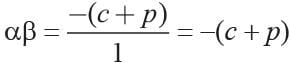Now (α + 1) (β+ 1) = αβ + α + β + 1 = - c -p + p + 1 = 1 - c

Math Olympiad Test: Quadratic Equations- 2 - Question 12

If the difference of the root x2 -px + q = 0 is unity then

Detailed Solution for Math Olympiad Test: Quadratic Equations- 2 - Question 12

We have x2 - px + q = 0
Now α + β = p, αβ = q
∴ α - β = 1
2α = p + 1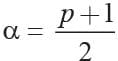Now
β = p - α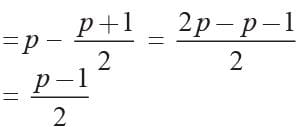and     αβ = q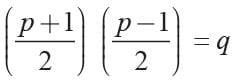p2 - 1 = 4q ⇒ p2 - 4q = 1

Math Olympiad Test: Quadratic Equations- 2 - Question 13

If a, b are the roots of the equation x2 + x + 1 = 0 then a2 + b2 = ?

Detailed Solution for Math Olympiad Test: Quadratic Equations- 2 - Question 13

Given a and b are roots of x2 + x + 1 = 0
then a + b = -1 and ab = 1
∴ a2 + b2 = (a + b)2 - 2ab
= (-1)2 - 2(1) = 1 - 2 = -1

Math Olympiad Test: Quadratic Equations- 2 - Question 14

If A and B are the roots of the quadratic equation x2 - 12x + 27 = 0, then A3 + B3 is

Detailed Solution for Math Olympiad Test: Quadratic Equations- 2 - Question 14

Given A and B are the roots of x2 - 12x + 27 = 0
⇒ (x - 3)(x - 9) = 0
∴ A =  3, B = 9
Hence A3 + B3 = 33 + 93 = 27 + 729 = 756

Math Olympiad Test: Quadratic Equations- 2 - Question 15

If the quadratic equation (a2 - b2)x2 + (b2 - c2)x + (c2 - a2) = 0 has equal roots, then which of the following is true?

Detailed Solution for Math Olympiad Test: Quadratic Equations- 2 - Question 15

If the quadratic equation is given by Ax2 + Bx + C = 0.
Then,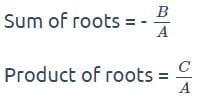Given: (a2 - b2)x2 + (b2 - c2)x + (c2 - a2) = 0
Where,
A = (a2 - b2), B = (b2 - c2), C = c2 - a2
Calculation:
Here,
A + B + C = (a2 - b2) + (b2 - c2) + (c2 - a2) = 0.
So, we can say that x = 1 is a root of a given quadratic equation. According to question both roots are same.
Then,
Roots (x) = 1, 1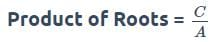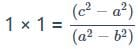(a2 - b2) = (c- a2)
By arranging, we get
b2 + c2 = 2a2

## International Mathematics Olympiad (IMO) for Class 10

22 docs|62 tests
 Use Code STAYHOME200 and get INR 200 additional OFF Use Coupon Code